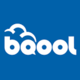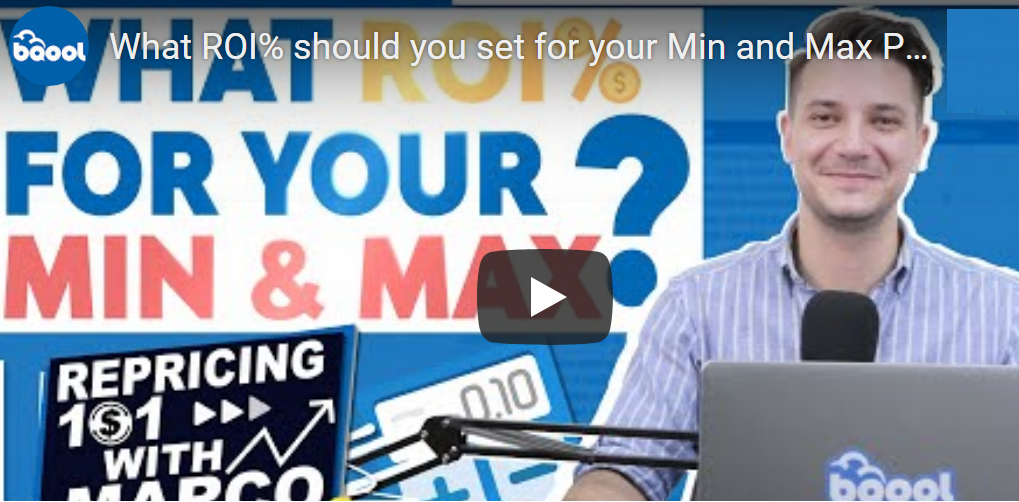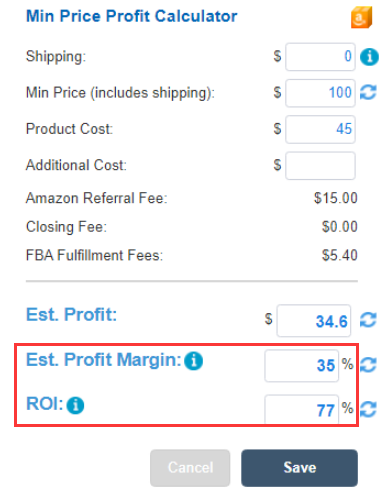# Estimated Profit Margin % and ROIBQool Support
• Updated

# Profit Calculator’s Estimated Profit Margin % and Return on Cost of Goods Sold

BQool will explain what is the ROI% and how you can use this calculation to set perfect Min and Max prices using a repricer. We will go through some real products and calculate the best ROI in order to prevent selling for a loss. Lastly, Marco will show how to successfully set the same ROI for multiple listings by using Bulk Action: Profits, ROI and Profit Margin feature.There are two common ways to estimate profitability in the business world – one is to consider the profit margin, and the other is to calculate Return on Investment. Profit margin % is calculated by breaking down the item price into cost and profit, whereas ROI focuses on the investment value of a product.

• Est. Profit Margin % is calculated based on the offer price (Min or Max)

Our current formula: Est. Profit Margin % = Profit (offer price – total cost) / Sale Price

Example: You purchased an item for \$45, and it was sold at \$100. After taking into account the product cost (\$45), assumed FBA fee of US\$10, and 15% Amazon Referral Fee (\$15), your net profit margin is 30%, which is calculated by \$30 / \$100.

Now if you were able to sell the product at \$500, your profit % will NOT be 150% (30% x 5). Since the Amazon Commission (%) is based on the Selling Price, the higher the price the greater the fee you need to pay to Amazon. Thus, your new cost is now \$130 (product cost \$45 + FBA fee of US\$10 + commission \$75). The new Profit % is now 74% ((\$500 – 130) / \$500).

• Return on cost of investment (ROI)

If you wish to set your Min or Max price based on a percentage of your actual cost, then you are looking to calculate ROI, or in other words, Markup % or Returns on the Cost of Goods Sold (COGS).

FormulaMarkup % = (Est. Profit  / Product Cost＋Additional Cost) X 100

Example: You bought a product at \$45 and you decided to price the item at \$100. If you do not consider all other cost, the Return on the \$45 is 122.2% { (\$55 / \$45 ) X 100 }.

In summary

In both examples the product was sold at \$100 and purchased at \$45

Est. Profit Margin % = Est. Profit (offer price – total cost) / Sale Price =

(Min Price \$100 - Product Cost \$45 - Amazon Referral Fee \$15 - FBA Fulfillment Fees \$5.40 ) / Min Price \$100 = \$34.60 / \$100 = 34.6%= 35%

ROI = (Est. Profit  / Product Cost+ Additional Cost) X 100 = (\$34.60 / Product Cost \$45 ) X 100= 76.88% = 77%8 out of 11 found this helpful